variables## variables

بازدید : 572 جمعه 12 دي 1393

Variable Names
Defining New Variables
Undefining Variables

## Variable names

The complete specification for a variable name is:

`abbrev.file#(dimexpr,dimexpr,...)`    where:

`abbrev   `is the abbreviation for the variable as specified in the data descriptor file
``` file#    is the file number that contains this variable. If file# is absent, grads assumes the default file number.  ``` ``` dimexpr  is a dimension expression that locally modifies the current dimension environment. ```

The `dimexpr` is used to override or modify the current dimension environment only for the `abbrev` variable. Only fixed dimensions can be modified with a `dimexpr`. The `dimexpr` can be given in grid or world coordinates. An important note: When you specify a dimension in grid units, grads always converts it to world coordinates. In general, this conversion is done using the scaling of the default file. The only exception to this rule is in this case, when a grid coordinate (x, y, z, t, or e) is supplied within a `dimexpr` as part of a variable specification. In the case of this special exception, the conversion is done using the scaling for the file that variable is to be taken from (i.e., file number `file#`).

An absolute `dimexpr` uses the "=" operator to override the currently set dimension value:
```   x|y|z|t|e|lon|lat|lev|time|ens = value ```
Examples of absolute dimension expressions are:` x=1, lat=-10, lev=500, t=1, time=02feb1982, e=1, ens=spr`

A relative `dimexpr` uses the "+" or "-" operators to modify the currently set dimension value with an offset:
```    x|y|z|t|e|lon|lat|lev|time|ens +/- offset ```
Examples of relative dimension expressions are:` x+1, lat+30, y-3, t+0, time+12hr`

Starting with grads version 2.0.a7, a third option is available, a `dimexpr` that specifies an offset from the variable's initial time:
```    offt =/+/- offset ```
Examples of absolute offset dimension expressions are:` offt=0, offt+4, offt-1`
Please consult the section on evaluating expressions that contain the `offt` dimension override for additional information.

The following sets of commands will yeild the same result, which is to display the 2nd time step of a variable "ps":
```   'set t 2'; 'd ps'   'set t 1'; 'd ps(t+1)'   'set t 1'; 'd ps(t=2)'   'set t 1'; 'd ps(offt=1)'   'set t 1'; 'd ps(offt+0)' ```
Note that an offset of 0 returns the 1st time step, an offset of 1 returns the 2nd time step, etc.

Using a `dimexpr` that contains `offt` is especially handy when your data set has multiple ensemble members, and the start time for individual members is not the same. In that case, an expression like:
```   'set t 1'; 'set e 1 last'; 'd ps(offt=0)' ```
will give you the first time step of all ensemble members, which may occur at different time steps in the file.

Examples of complete variable specifications are:

`z.3(lev=500)`          File 3, absolute dimension expression
``` tv.1(time-12hr)    Relative dimension expression ``` ``` rh                              Default file number is used  ``` ``` q.2(t-1,lev=850)  Two dimension expressions  ``` ``` z(t+0)                      This does have uses.... ```

grads has a few "predefined" variable names. You can think of these as being variables implicitly contained within any opened gridded file. The variable names are:

lat
``` lon  lev ```

When used, they will contain the `lat`, `lon`, and `lev` at the respective grid points, using the scaling of the appropriate file. You can specify: `lat.2` for example, to get latitudes on the grid of the 2nd opened data set.

## Defining new variables

The `define` command allows you to interactively create a new variable. The syntax is:

`define varname = expression`

The new variable can then be used in subsequent `define` and/or `display` commands. The new variable is stored in memory, not on disk, so avoid defining variables over large dimension ranges.

Defined variables cover the dimension ranges in effect at the time the command is issued. You may define a variable that has from 0 to 4 varying dimensions. The `define` command is the only case within GrADS where four varying dimensions is valid.

When `Z` and/or `T` are varying dimensions, the `define` command evaluates the expression by stepping through `Z` and `T`. In other words, the expression is evaluated within a dimension environment that has fixed `Z` and `T`. This will affect how you compose the expression.

When you use a defined variable, data is taken from the variable in a way similar to data taken from a GrADS data file. For example, say you define a four dimensional variable:

set lon -180 0
``` set lat 0 90  set lev 1000 100  set t 1 10  define temp = rh ```

After issuing the `define` command, remember to change the dimension environment so less than 4 dimensions are varying!

set t 5
``` set lev 500  d temp ```

The display of the defined variable will display a 2-D slice taken at time 5 and level 500.

If you define a variable that has fixed dimensions, and then later access this variable, the fixed dimensions are treated as "wild cards". The best way to show this is with an example:

set lon -180 0
``` set lat 0 90  set lev 500  set t 10  define zave = ave(z,t=1,t=30) ```

The defined variable has two varying dimensions. If we now display this variable (or use it in an expression), the fixed dimensions of the defined variable, namely `Z` and `T`, will match ANY `Z` and `T` dimension setting:

set t 1
``` set lev 200  d zave ```

In the above display, the variable `zave` would be displayed as it was defined, ie you would get a time average of 500mb heights, even though the level is set to 850.

When the defined variable has varying dimensions, and you have a dimension environment where that dimension is fixed, the proper dimension will be retrieved from the variable:

set lon -180 0
``` set lat 0 90  set lev 500  set t 10  define temp = z set lat 40  d temp ```

In the above example, the defined variable has a varying Y dimension. We then fix the Y dimension to be 40N, and display a 1-D slice. The data from 40N in the defined grid will be accessed. If you then did:

set lat -40
``` d temp ```

The data from 40S would be accessed from the defined variable. Since this is beyond the dimensions originally used when the variable was defined, the data would be set to missing.

You can also locally override the dimension environment:

`d temp(lat=50)`

If that dimension is a varying dimension within the defined variable. If the dimension is a fixed dimension for that variable, the local override will be ignored:

`d temp(t=15)`

In the above command, the defined variable temp has fixed T, so the t=15 would be ignored.

N.B.: The `define` command currently supports only grids.

Once you have defined a grid variables, you may tell GrADS that the new variable is climatological, ie that you wish to treat the time dimension of the new variable in a wild card sense.

The command is:

``` modify varname <seasonal/diurnal> ```

where `varname` is the name of a defined variable. If the grid is described as `seasonal`, then it is assumed that the defined variable contains monthly (or multi month) means. Daily or multi-day means are not yet supported. If `diurnal` is specified, it is assumed the defined variable contains means over some time period less than a day.

After describing the defined variable as climatological, then the date/times are treated appropriately when data is accessed from the defined variable.

In the following example, the data set contains 10 years of monthly means:

set lon -180 180
``` set lat -90 90  set lev 500  set t 1 12  define zave = ave(z,t+0,t=120,1yr) ```

This define will set up a variable called `zave` which contains 12 times, each time being the 10 year mean for that month. We are making use here of the fact that the define command loops through a varying time dimension when evaluating the expression, and within the `ave` function we are making use of the variable time offset of t+0, which uses a start time that is whatever time the `define` command is using as it loops.

modify zave seasonal
``` set t 120  d z - zave ```

The final display will remove the 10 year monthly mean for December from the last December in the data set.

## Undefining variables

Each variable defined using the `define` command reserves some system resources. If you no longer need a defined variable it is sensible to free these resources for other use. This is accomplished with the `undefine` command. For example:

`undefine p`

would free the resources used by the defined variable `p`. Of course, the variable `p` would no longer be available for GrADS processing.

مطالب مرتبط
ارسال نظر برای این مطلب

نام
ایمیل (منتشر نمی‌شود) (لازم)
وبسایت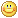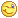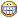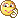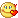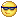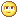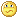نظر خصوصی
مشخصات شما ذخیره شود ؟ [حذف مشخصات] [شکلک ها]کد امنیتی
اطلاعات کاربری
 نام کاربری : رمز عبور :
• فراموشی رمز عبور؟
• لینک دوستان
آرشیو
آمار سایت
• کل مطالب : 155
• کل نظرات : 0
• افراد آنلاین : 1
• تعداد اعضا : 4
• آی پی امروز : 5
• آی پی دیروز : 17
• بازدید امروز : 51
• باردید دیروز : 81
• گوگل امروز : 0
• گوگل دیروز : 1
• بازدید هفته : 295
• بازدید ماه : 267
• بازدید سال : 36,441
• بازدید کلی : 157,493
• کدهای اختصاصی